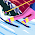Electrical Load Calculator for Dwelling Units

I explained Where and how to distribute each type of load in a dwelling unit as per NEC code. Also I explained how to calculate the Demand load for each type of dwelling loads for feeder and service sizing calculations in the following Articles:

Today, I introduce to you, the most professional Excel Sheet for calculating the Total Demand Load for single, Two-Family, Multi-Family Dwelling Units as per NEC Standard Calculation Method.

This excel Sheet is exclusive for our website Electrical Know how, You will not find this Professional excel sheet anywhere in the web.

the benefits of using this sheet than other similar ones are as follows:

• it explains all the NEC rules applied for each calculation step inside the sheet.
• It is the most applicable calculator for all types of dwelling units; single, two-family and multi-family.
• It is the easy one for understanding and application.
• Some Demand Factors Tables are included in the excel sheet and no need to manually extract its data from the code as other similar ones do.

I put the excel sheet in a word format in below just for easy reviewing for each step calculations, rules, exceptions and tables.

 TOTAL DEMAND LOAD = #DIV/0! VA

in the next Article I will introduce Electrical Load Calculator for Dwelling Units as per NEC Optional Method. please, keep following.

1.بارك الله فيك م علي

2.Thanks Ali so Much!

3.الف شكر باش مهندس على